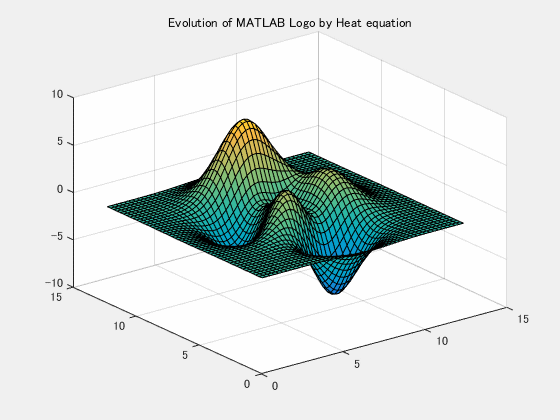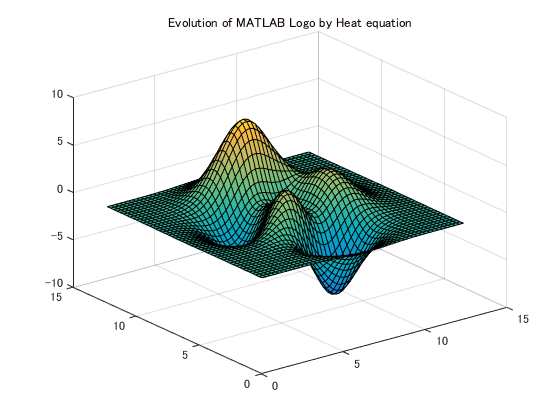## Simple Heat Equation solver

Version 1.0.0.3 (5.51 MB) by
Simple Heat Equation solver using finite difference method

Updated 18 Mar 2023

From GitHub

# Finite differences for the 2D heat equation

Implementation of a simple numerical schemes for the heat equation.Applying the second-order centered differences to approximate the spatial derivatives,Neumann boundary condition is employed for no-heat flux, thus please note that the grid location is staggered. Once the right hand side is obtained, the equation can be solved by the ODE suite. Here we use ode15s. Copyright 2015-2016 The MathWorks, Inc.# Problem Setup

N = 50; % Number of grid in x,y-direction
L = 4*pi; % Domain size

% Grid point
x = linspace(0,L,N);
y = linspace(0,L,N);
% Make it staggered.
x = (x(1:end-1)+x(2:end))/2;
y = (y(1:end-1)+y(2:end))/2;
[X,Y] = meshgrid(x,y);

# Initial Condition

% Let's use MATLAB logo.
% A variable u0 is defined at the center of each grid cell
% thus the number of grid point is N-1.
u0(:,:) = peaks(N-1);

% Plot it
handle_surf = surf(X,Y,u0);
handle_axes = gca;
handle_axes.ZLim = [-10,10];
handle_axes.CLim = [-10,10];
title('Evolution of MATLAB Logo by Heat equation');# Simulation

dx = x(2)-x(1); % spatial grid size
alpha = 2; % coefficient
tspan = linspace(0,1,40);
[t,u] = ode15s(@(t,x)getRHS(x,alpha,dx,N),tspan,u0(:));

# Visualize

Tn = length(t);
u = reshape(u,Tn,N-1,N-1);

filename = 'heat.gif';
for ii=1:Tn
Z = u(ii,:,:);
Z = squeeze(Z);
handle_surf.ZData = Z;
drawnow;
frame = getframe(gcf);
im = frame2im(frame);
[A,map] = rgb2ind(im,256);
if ii==1
imwrite(A,map,filename,'gif','LoopCount',Inf,'DelayTime',0.05);
else
imwrite(A,map,filename,'gif','WriteMode','append','DelayTime',0.05);
end
end### Cite As

michio (2023). Simple Heat Equation solver (https://github.com/mathworks/Simple-Heat-Equation-solver), GitHub. Retrieved .

##### MATLAB Release Compatibility
Created with R2016a
Compatible with any release
##### Platform Compatibility
Windows macOS Linux
##### Categories
Find more on PDE Solvers in Help Center and MATLAB Answers

### Community Treasure Hunt

Find the treasures in MATLAB Central and discover how the community can help you!

Start Hunting!

Version Published Release Notes
1.0.0.3

reflect markdown on File Exchange

1.0.0.2

Move to GitHub

1.0.0.1